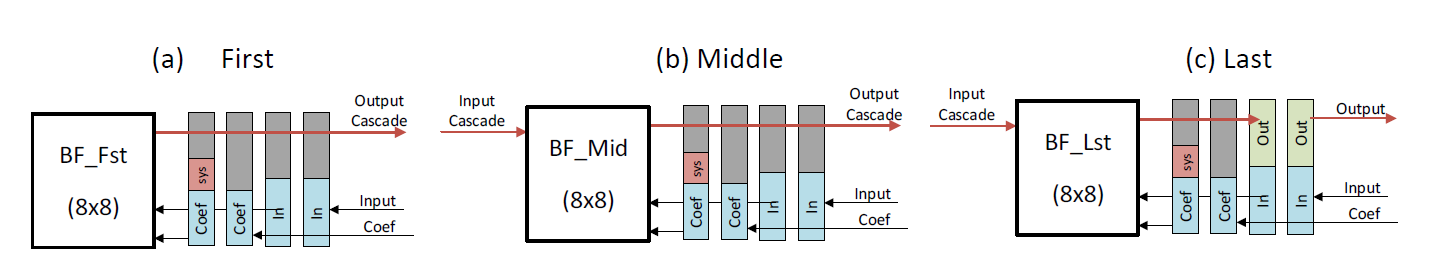# AI Engine Kernels and Graphs - 2023.2 English

## Vitis Tutorials: AI Engine

Document ID
XD100
Release Date
2023-11-29
Version
2023.2 English

The fundamental beamforming kernel computes a complex matrix multiplication on a (8x8) coefficient matrix times a (8x1) input data matrix which results in a (8x1) output data matrix. The beamforming kernel repeats the matrix multiplication computation on as many (8x1) input data matrices as are fed into it.

When the beamforming kernel computes the matrix multiplication, the results are only partial summations. The beamforming kernel then sends the partial results to identical kernels in a cascade chain for further accumulation and multiplication into a final accumulated (8x1) output data matrix. A real 5G system is determined by the number of layers (M) and number of antennas (N). For this beamforming tutorial, M is determined by the number of inputs into the chain (that is, the length of the input data matrix (8) times the number of kernels in a cascade chain). N is determined by the number of final accumulated outputs at the end of the chain (8) times the number of chains in your design.

This beamforming kernel comes in three flavors: `bf8x8_fst`, `bf8x8_mid`, and `bf8x8_lst`. Review the source files defined in the `src/kernels/` folder.The kernels are strung together into a cascading chain, and they are named first, middle, and last depending on their location in the cascading chain. All three of these kernels implement the matrix multiplication function and only differ in the input and output interfaces. The first kernel in the cascading chain does not have a cascading input because it is the first kernel in a chain. The middle kernel has both a cascading input and a cascading output. The last kernel has both a cascading input and output, but writes the output to local memory instead of the cascading bus.

All three beamforming kernel types start by loading the input data and input coefficient data from PL kernels. The first beamforming kernel computes the matrix multiplication, computes the first partial summation, and sends it to a middle kernel using an output cascade. The middle kernel reads the partial summation from the previous kernel, computes its own matrix multiplication on the accumulated data, and generates its own output cascade. This output cascade becomes the input cascade to either another middle kernel or the last kernel. The last kernel does the final matrix multiplication on the accumulated data and writes the final results to a PL kernel. The entire chain of beamforming kernels repeats these calculations on new input data for 12 subcarriers in total.